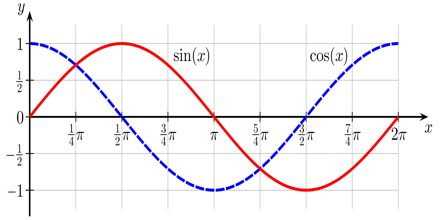Mathematic

# Graphing Sine and CosinePrinciple purpose of this lecture is to present on Graphing Sine and Cosine. Sine, Cosine and Tangent are the main functions used in Trigonometry and are based on a Right-Angled Triangle. Graphs that repeat infinitely in intervals are called periodic. Example: Sea level as a function of time. The most common periodic graphs are the graphs of the trigonometric functions Sine, cosine, tangent, cosecant, secant, and cotangent. The cosine graph is the same as the sine graph, only it has been shifted horizontally.